# Active Matter¶

## Introduction¶

In this tutorial we explore the ways to simulate self-propulsion in the simulation software package ESPResSo. We consider three examples that illustrate the properties of these systems. First, we study the concept of enhanced diffusion of a self-propelled particle. Second, we investigate rectification in an asymmetric geometry. Finally, we determine the flow field around a self-propelled particle using lattice-Boltzmann simulations (LB). These three subsections should give insight into the basics of simulating active matter with ESPResSo. This tutorial assumes basic knowledge of Python and ESPResSo, as well as the use of lattice-Boltzmann within ESPResSo. It is therefore recommended to go through the relevant tutorials first, before attempting this one.

## Active particles¶

Active matter is a term that describes a class of systems, in which energy is constantly consumed to perform work. These systems are therefore highly out-of-equilibrium (thermodynamically) and (can) thus defy description using the standard framework of statistical mechanics. Active systems are, however, ubiquitous. On our length scale, we encounter flocks of birds, schools of fish, and, of course, humans; on the mesoscopic level examples are found in bacteria, sperm, and algae; and on the nanoscopic level, transport along the cytoskeleton is achieved by myosin motors. This exemplifies the range of length scales which the field of active matter encompasses, as well as its diversity. Recent years have seen a huge increase in studies into systems consisting of self-propelled particles, in particular artificial ones in the colloidal regime. These self-propelled colloids show promise as physical model systems for complex biological behavior (bacteria moving collectively) and could be used to answer fundamental questions concerning out-of-equilibrium statistical physics. Simulations can also play an important role in this regard, as the parameters are more easily tunable and the results ‘cleaner’ than in experiments. The above should give you some idea of the importance of the field of active matter and why you should be interested in performing simulations in it.

### Active Particles in ESPResSo¶

The ENGINE feature offers intuitive syntax for adding self-propulsion to a particle. The propulsion will occur along the vector that defines the orientation of the particle (henceforth referred to as ‘director’). In ESPResSo the orientation of the particle is defined by a quaternion; this in turn defines a rotation matrix that acts on the particle's initial orientation (along the z-axis), which then defines the particles current orientation through the matrix-oriented vector. Within the ENGINE feature there are two ways of setting up a self-propelled particle, with and without hydrodynamic interactions. The particle without hydrodynamic interactions will be discussed first, as it is the simplest case.

### Self-Propulsion without Hydrodynamics¶

For this type of self-propulsion the Langevin thermostat can be used. The Langevin thermostat imposes a velocity-dependent friction on a particle. When a constant force is applied along the director, the friction causes the particle to attain a terminal velocity, due to the balance of driving and friction force, see Fig. 1. The exponent with which the particle's velocity relaxes towards this value depends on the strength of the friction and the mass of the particle. The ENGINE feature implies that rotation of the particles (the ROTATION feature) is compiled into ESPResSo. The particle can thus reorient due to external torques or due to thermal fluctuations, whenever the rotational degrees of freedom are thermalized. Note that the rotation of the particles has to be enabled explicitly via their ROTATION property. This ‘engine’ building block can be connected to other particles, e.g., via the virtual sites (rigid body) to construct complex self-propelled objects.Fig. 1: A balance of the driving force in the direction defined by the ‘director’ unit vector and the friction due to the Langevin thermostat results in a constant terminal velocity.

## Enhanced Diffusion¶

First we import the necessary modules, define the parameters and set up the system.

In :
%matplotlib inline
import matplotlib.pyplot as plt

In :
plt.rcParams.update({'font.size': 18})

import tqdm
import numpy as np
import espressomd.observables
import espressomd.accumulators

espressomd.assert_features(
["ENGINE", "ROTATION", "MASS", "ROTATIONAL_INERTIA", "CUDA"])

In :
ED_PARAMS = {'time_step': 0.01,
'box_l': 3*[10.],
'skin': 0.4,
'active_velocity': 5,
'kT': 1,
'gamma': 1,
'gamma_rotation': 1,
'mass': 0.1,
'rinertia': 3*[1.],
'corr_tmax': 100}
ED_N_SAMPLING_STEPS = 5000000

In :
system = espressomd.System(box_l=ED_PARAMS['box_l'])
system.cell_system.skin = ED_PARAMS['skin']
system.time_step = ED_PARAMS['time_step']


### Exercise¶

In :
system.thermostat.set_langevin(kT=ED_PARAMS['kT'],
gamma=ED_PARAMS['gamma'],
gamma_rotation=ED_PARAMS['gamma_rotation'],
seed=42)


The configuration for the Langevin-based swimming is exposed as an attribute of the ParticleHandle class of ESPResSo, which represents a particle in the simulation. You can either set up the self-propulsion during the creation of a particle or at a later stage.

### Exercise¶

• Set up one active and one passive particle, call them part_act and part_pass (Hint: see the docs)
• Use ED_PARAMS for the necessary parameters
In :
part_act = system.part.add(pos=[5.0, 5.0, 5.0], swimming={'v_swim': ED_PARAMS['active_velocity']},
mass=ED_PARAMS['mass'], rotation=3 * [True], rinertia=ED_PARAMS['rinertia'])
mass=ED_PARAMS['mass'], rotation=3 * [True], rinertia=ED_PARAMS['rinertia'])


Next we set up three ESPResSo correlators for the Mean Square Displacement (MSD), Velocity Autocorrelation Function (VACF) and the Angular Velocity Autocorrelation Function (AVACF).

In :
pos_obs = espressomd.observables.ParticlePositions(
ids=[part_act.id, part_pass.id])
msd = espressomd.accumulators.Correlator(obs1=pos_obs,
corr_operation="square_distance_componentwise",
delta_N=1,
tau_max=ED_PARAMS['corr_tmax'],
tau_lin=16)

vel_obs = espressomd.observables.ParticleVelocities(
ids=[part_act.id, part_pass.id])
vacf = espressomd.accumulators.Correlator(obs1=vel_obs,
corr_operation="componentwise_product",
delta_N=1,
tau_max=ED_PARAMS['corr_tmax'],
tau_lin=16)

ang_obs = espressomd.observables.ParticleAngularVelocities(
ids=[part_act.id, part_pass.id])
avacf = espressomd.accumulators.Correlator(obs1=ang_obs,
corr_operation="componentwise_product",
delta_N=1,
tau_max=ED_PARAMS['corr_tmax'],
tau_lin=16)


No more setup needed! We can run the simulation and plot our observables.

In :
for i in tqdm.tqdm(range(100)):
system.integrator.run(int(ED_N_SAMPLING_STEPS/100))

100%|██████████| 100/100 [00:59<00:00,  1.68it/s]

In :
system.auto_update_accumulators.remove(msd)
msd.finalize()
system.auto_update_accumulators.remove(vacf)
vacf.finalize()
system.auto_update_accumulators.remove(avacf)
avacf.finalize()

In :
taus_msd = msd.lag_times()
msd_result = msd.result()
msd_result = np.sum(msd_result, axis=2)

taus_vacf = vacf.lag_times()
vacf_result = np.sum(vacf.result(), axis=2)

taus_avacf = avacf.lag_times()
avacf_result = np.sum(avacf.result(), axis=2)

In :
fig_msd = plt.figure(figsize=(10, 6))
plt.plot(taus_msd, msd_result[:, 0], label='active')
plt.plot(taus_msd, msd_result[:, 1], label='passive')
plt.xlim((taus_msd, None))
plt.loglog()
plt.xlabel('t')
plt.ylabel('MSD(t)')
plt.legend()
plt.show()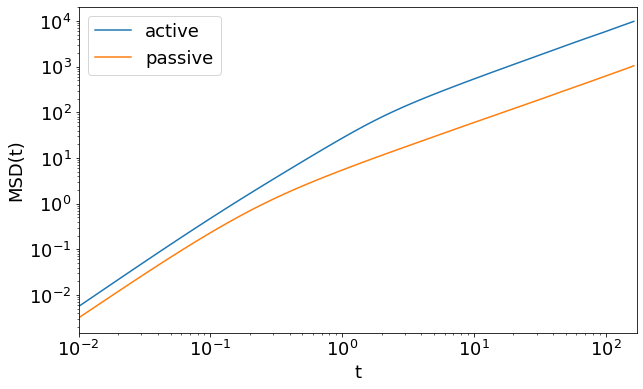The Mean Square Displacement of an active particle is characterized by a longer ballistic regime and an increased diffusion coefficient for longer lag times. In the overdamped limit it is given by

$$\langle r^{2}(t) \rangle = 6 D t + \frac{v^{2} \tau^{2}_{R}}{2} \left[ \frac{2 t}{\tau^{2}_{R}} + \exp\left( \frac{-2t}{\tau^{2}_{R}} \right) - 1 \right],$$

where $\tau_{R} = \frac{8\pi\eta R^{3}}{k_{B} T}$ is the characteristic time scale for rotational diffusion and $D = \frac{k_B T}{\gamma}$ is the translational diffusion coefficient.

For small times ($t \ll \tau_{R}$) the motion is ballistic

$$\langle r^{2}(t) \rangle = 6 D t + v^{2} t^{2},$$

while for long times ($t \gg \tau_{R}$) the motion is diffusive

$$\langle r^{2}(t) \rangle = (6 D + v^{2}\tau_{R}) t.$$

Note that no matter the strength of the activity, provided it is some finite value, the crossover between ballistic motion and enhanced diffusion is controlled by the rotational diffusion time.

The passive particle also displays a crossover from a ballistic to a diffusive motion. However, the crossover time $\tau_{C}=\frac{m}{\gamma}$ is not determined by the rotational motion but instead by the mass of the particles.

From the longterm MSD of the active particles we can define an effective diffusion coefficient $D_{\mathrm{eff}} = D + v^{2}\tau_{R}/6$. One can, of course, also connect this increased diffusion with an effective temperature. However, this apparent equivalence can lead to problems when one then attempts to apply statistical mechanics to such systems at the effective temperature. That is, there is typically more to being out-of-equilibrium than can be captured by a simple remapping of equilibrium parameters, as we will see in the second part of the tutorial.

From the autocorrelation functions of the velocity and the angular velocity we can see that the activity does not influence the rotational diffusion. Yet the directed motion for $t<\tau_{R}$ leads to an enhanced correlation of the velocity.

In :
def acf_stable_regime(x, y):
"""
Remove the noisy tail in autocorrelation functions of finite time series.
"""
cut = np.argmax(y <= 0.) - 2
assert cut >= 1
return (x[1:cut], y[1:cut])

In :
fig_vacf = plt.figure(figsize=(10, 6))
plt.plot(*acf_stable_regime(taus_vacf, vacf_result[:, 0]), label='active')
plt.plot(*acf_stable_regime(taus_vacf, vacf_result[:, 1]), label='passive')
plt.xlim((taus_vacf, None))
plt.loglog()
plt.xlabel('t')
plt.ylabel('VACF(t)')
plt.legend()
plt.show()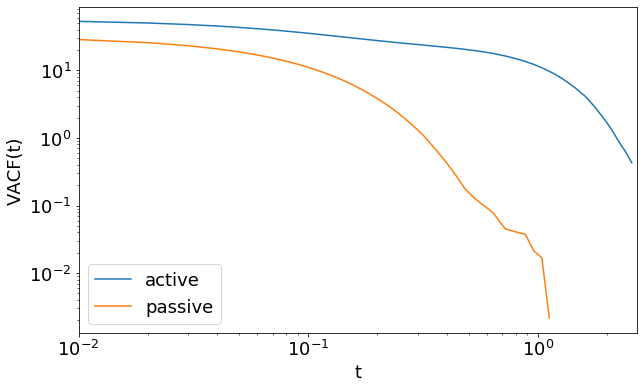In :
fig_avacf = plt.figure(figsize=(10, 6))
plt.plot(*acf_stable_regime(taus_avacf, avacf_result[:, 0]), label='active')
plt.plot(*acf_stable_regime(taus_avacf, avacf_result[:, 1]), label='passive')
plt.xlim((taus_avacf, None))
plt.loglog()
plt.xlabel('t')
plt.ylabel('AVACF(t)')
plt.legend()
plt.show()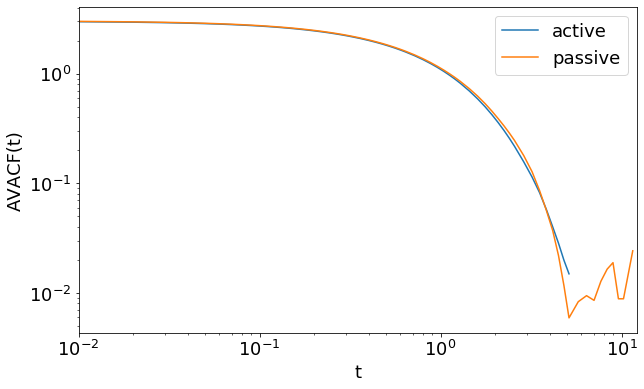Before we go to the second part, it is important to clear the state of the system.

In :
def clear_system(system):
system.part.clear()
system.thermostat.turn_off()
system.constraints.clear()
system.auto_update_accumulators.clear()
system.time = 0.

In :
clear_system(system)


## Rectification¶

In the second part of this tutorial you will consider the ‘rectifying’ properties of certain asymmetric geometries on active systems. Rectification can be best understood by considering a system of passive particles first. In an equilibrium system, for which the particles are confined to an asymmetric box with hard walls, we know that the particle density is homogeneous throughout. However, in an out-of-equilibrium setting one can have a heterogeneous distribution of particles, which limits the applicability of an ‘effective’ temperature description.

The geometry we will use is a cylindrical system with a funnel dividing two halves of the box as shown in Fig. 2.Fig. 2: Sketch of the rectifying geometry which we simulate for this tutorial.
In :
import espressomd.shapes
import espressomd.math

In :
RECT_PARAMS = {'length': 100,
'funnel_angle': np.pi / 4.0,
'funnel_thickness': 0.1,
'n_particles': 500,
'active_velocity': 7,
'time_step': 0.01,
'wca_sigma': 0.5,
'wca_epsilon': 0.1,
'skin': 0.4,
'kT': 0.1,
'gamma': 1.,
'gamma_rotation': 1}

RECT_STEPS_PER_SAMPLE = 100
RECT_N_SAMPLES = 500

TYPES = {'particles': 0,
'boundaries': 1}

box_l = np.array(
system.box_l = box_l
system.cell_system.skin = RECT_PARAMS['skin']
system.time_step = RECT_PARAMS['time_step']
system.thermostat.set_langevin(
kT=RECT_PARAMS['kT'], gamma=RECT_PARAMS['gamma'], gamma_rotation=RECT_PARAMS['gamma_rotation'], seed=42)

In :
cylinder = espressomd.shapes.Cylinder(
center=0.5 * box_l,

# Setup walls
wall = espressomd.shapes.Wall(dist=0, normal=[1, 0, 0])

wall = espressomd.shapes.Wall(dist=-RECT_PARAMS['length'], normal=[-1, 0, 0])

Out:
<espressomd.constraints.ShapeBasedConstraint at 0x7f3cb00f0900>
In :
funnel_length = (RECT_PARAMS['radius']-RECT_PARAMS['funnel_inner_radius']
)/np.tan(RECT_PARAMS['funnel_angle'])


### Exercise¶

• Using funnel_length and the geometric parameters in RECT_PARAMS, set up the funnel cone (Hint: Conical Frustum)
In :
ctp = espressomd.math.CylindricalTransformationParameters(
axis=[1, 0, 0], center=box_l/2.)

hollow_cone = espressomd.shapes.HollowConicalFrustum(
cyl_transform_params=ctp,
thickness=RECT_PARAMS['funnel_thickness'],
length=funnel_length,
direction=1)

Out:
<espressomd.constraints.ShapeBasedConstraint at 0x7f3cb0081810>

### Exercise¶

• Set up a WCA potential between the walls and the particles using the parameters in RECT_PARAMS
In :
system.non_bonded_inter[TYPES['particles'], TYPES['boundaries']].wca.set_params(
epsilon=RECT_PARAMS['wca_epsilon'], sigma=RECT_PARAMS['wca_sigma'])


ESPResSo uses quaternions to describe the rotational state of particles. Here we provide a convenience method to calculate quaternions from spherical coordinates.

### Exercise¶

• Place an equal number of swimming particles (the total number should be RECT_PARAMS['n_particles']) in the left and the right part of the box such that the center of mass is exactly in the middle. (Hint: Particles do not interact so you can put multiple in the same position)
• Particles must be created with a random orientation
In :
for i in range(RECT_PARAMS['n_particles']):
pos = box_l / 2
pos += (-1)**i * 0.25 * RECT_PARAMS['length']

# https://mathworld.wolfram.com/SpherePointPicking.html
theta = np.arccos(2. * np.random.random() - 1)
phi = 2. * np.pi * np.random.random()
director = [np.sin(theta) * np.cos(phi),
np.sin(theta) * np.cos(phi),
np.cos(theta)]

director=director, rotation=3*[True])

In :
com_deviations = list()
times = list()


### Exercise¶

• Run the simulation using RECT_N_SAMPLES and RECT_STEPS_PER_SAMPLE and calculate the deviation of the center of mass from the center of the box in each sample step. (Hint: Center of mass)
• Save the result and the corresponding time of the system in the lists given above.
In :
for _ in tqdm.tqdm(range(RECT_N_SAMPLES)):
system.integrator.run(RECT_STEPS_PER_SAMPLE)
com_deviations.append(system.galilei.system_CMS() - 0.5 * box_l)
times.append(system.time)

100%|██████████| 500/500 [00:43<00:00, 11.47it/s]

In :
def moving_average(data, window_size):
return np.convolve(data, np.ones(window_size), 'same') / window_size

In :
smoothing_window = 10
com_smoothed = moving_average(com_deviations, smoothing_window)
fig_rect = plt.figure(figsize=(10, 6))
plt.plot(times[smoothing_window:-smoothing_window],
com_smoothed[smoothing_window:-smoothing_window])
plt.xlabel('t')
plt.ylabel('center of mass deviation')
plt.show()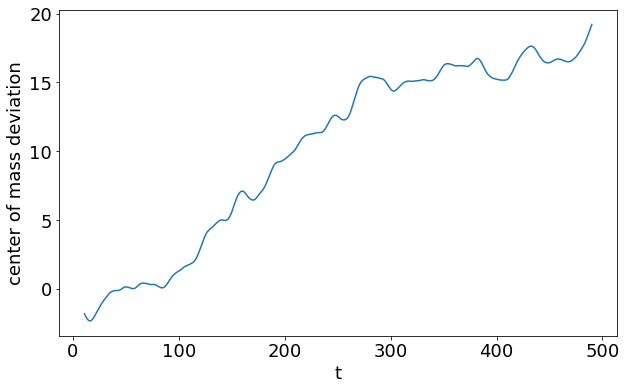Even though the potential energy inside the geometry is 0 in every part of the accessible region, the active particles are clearly not Boltzmann distributed (homogenous density). Instead, they get funneled into the right half, showing the inapplicability of equilibrium statistical mechanics.

In :
clear_system(system)


## Hydrodynamics of self-propelled particles¶

In situations where hydrodynamic interactions between swimmers or swimmers and objects are of importance, we use the lattice-Boltzmann (LB) to propagate the fluid's momentum diffusion. We recommend the GPU-based variant of LB in ESPResSo, since it is much faster. Moreover, the current implementation of the CPU self-propulsion is limited to one CPU. This is because the ghost-node structure of the ESPResSo cell-list code does not allow for straightforward MPI parallelization of the swimmer objects across several CPUs.

Of particular importance for self-propulsion at low Reynolds number is the fact that active systems (bacteria, sperm, algae, but also artificial chemically powered swimmers) are force free. That is, the flow field around one of these objects does not contain a monopolar (Stokeslet) contribution. In the case of a sperm cell, see Fig. 3(a), the reasoning is as follows. The whip-like tail pushes against the fluid and the fluid pushes against the tail, at the same time the head experiences drag, pushing against the fluid and being pushed back against by the fluid. This ensures that both the swimmer and the fluid experience no net force. However, due to the asymmetry of the distribution of forces around the swimmer, the fluid flow still causes net motion. When there is no net force on the fluid, the lowest-order multipole that can be present is a hydrodynamic dipole. Since a dipole has an orientation, there are two types of swimmer: pushers and pullers. The distinction is made by whether the particle pulls fluid in from the front and back, and pushes it out towards its side (puller), or vice versa (pusher), see Fig. 3(c,d).Fig. 3: (a) Illustration of a sperm cell modeled using our two-point swimmer code. The head is represented by a solid particle, on which a force is acting (black arrow). In the fluid a counter force is applied (white arrow). This generates a pusher-type particle. (b) Illustration of the puller-type Chlamydomonas algae, also represented by our two-point swimmer. (c,d) Sketch of the flow-lines around the swimmers: (c) pusher and (d) puller.

For the setup of the swimming particles with hydrodynamics we cannot use the v_swim argument anymore because it is not trivial to determine the friction acting on the particle. Instead, we have to provide the keys f_swim and dipole_length. Together they determine what the dipole strength and the terminal velocity of the swimmer is. One should be careful, however, the dipole_length should be at least one grid spacing, since use is made of the LB interpolation scheme. If the length is less than one grid spacing, you can easily run into discretization artifacts or cause the particle not to move. This dipole length together with the director and the keyword pusher/puller determines where the counter force on the fluid is applied to make the system force free, see Fig. 3(a) for an illustration of the setup. That is to say, a force of magnitude f_swim is applied to the particle (leading to a Stokeslet in the fluid, due to friction) and a counter force is applied to compensate for this in the fluid (resulting in an extended dipole flow field, due to the second monopole). For a puller the counter force is applied in front of the particle and for a pusher it is in the back (Fig. 3(b)).

Finally, there are a few caveats to the swimming setup with hydrodynamic interactions. First, the stability of this algorithm is governed by the stability limitations of the LB method. Second, since the particle is essentially a point particle, there is no rotation caused by the fluid flow, e.g., a swimmer in a Poiseuille flow. If the thermostat is switched on, the rotational degrees of freedom will also be thermalized, but there is still no contribution of rotation due to ‘external’ flow fields. It is recommended to use an alternative means of obtaining rotations in your LB swimming simulations. For example, by constructing a raspberry particle.

In :
import espressomd.lb

In :
HYDRO_PARAMS = {'box_l': 3*,
'time_step': 0.01,
'skin': 1,
'agrid': 1,
'dens': 1,
'visc': 1,
'gamma': 1,
'mass': 5,
'dipole_length': 2,
'active_force': 0.1,
'mode': 'pusher'}

HYDRO_N_STEPS = 2000

system.box_l = HYDRO_PARAMS['box_l']
system.cell_system.skin = HYDRO_PARAMS['skin']
system.time_step = HYDRO_PARAMS['time_step']
system.min_global_cut = HYDRO_PARAMS['dipole_length']


### Exercise¶

• Using HYDRO_PARAMS, set up a lattice-Boltzmann fluid and activate it as a thermostat (Hint: lattice-Boltzmann)
In :
lbf = espressomd.lb.LBFluidGPU(agrid=HYDRO_PARAMS['agrid'], dens=HYDRO_PARAMS['dens'],
visc=HYDRO_PARAMS['visc'], tau=HYDRO_PARAMS['time_step'])
system.thermostat.set_lb(LB_fluid=lbf, gamma=HYDRO_PARAMS['gamma'], seed=42)

In :
box_l = np.array(HYDRO_PARAMS['box_l'])
pos = box_l/2.
pos = -10.


### Exercise¶

• Using HYDRO_PARAMS, place particle at pos that swims in z-direction. The particle handle should be called particle.
In :
particle = system.part.add(
pos=pos, mass=HYDRO_PARAMS['mass'], rotation=3*[False],
swimming={'f_swim': HYDRO_PARAMS['active_force'],
'mode': HYDRO_PARAMS['mode'],
'dipole_length': HYDRO_PARAMS['dipole_length']})

In :
system.integrator.run(HYDRO_N_STEPS)

In :
vels = np.squeeze(lbf[:, int(system.box_l/2), :].velocity)
vel_abs = np.linalg.norm(vels, axis=2)

lb_shape = lbf.shape
xs, zs = np.meshgrid(np.linspace(0.5, box_l - 0.5, num=lb_shape),
np.linspace(0.5, box_l - 0.5, num=lb_shape))

fig_vels, ax_vels = plt.subplots(figsize=(10, 6))
im = plt.pcolormesh(vel_abs.T, cmap='YlOrRd')
plt.quiver(xs, zs, vels[:, :, 0].T, vels[:, :, 2].T, angles='xy', scale=0.005)
circ = plt.Circle(particle.pos_folded[[0, 2]], 0.5, color='blue')
ax_vels.set_aspect('equal')
plt.xlabel('x')
plt.ylabel('z')
cb = plt.colorbar(im, label=r'$|v_{\mathrm{fluid}}|$')
plt.show()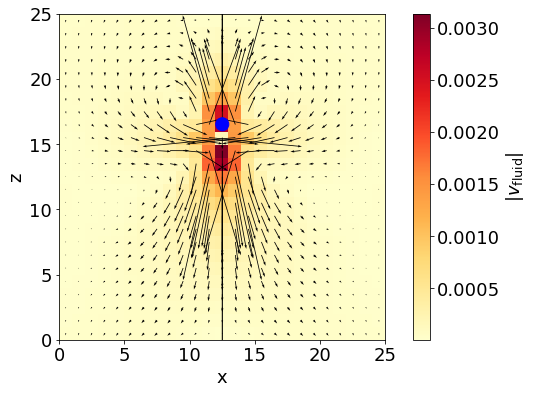We can also export the particle and fluid data to .vtk format to display the results with a visualization software like ParaView.

In :
lbf.write_vtk_velocity('./fluid.vtk')
system.part.writevtk('./particle.vtk')


The result of such a visualization could look like Fig. 4.Fig. 4: Snapshot of the flow field around a pusher particle visualized with ParaView.

 M. Ballerini, N. Cabibbo, R. Candelier, A. Cavagna, E. Cisbani, I. Giardina, V. Lecomte, A. Orlandi, G. Parisi, A. Procaccini, M. Viale, and V. Zdravkovic. Interaction ruling animal collective behavior depends on topological rather than metric distance: Evidence from a field study. Proc. Natl. Acad. Sci., 105:1232, 2008.
 Y. Katz, K. Tunstrøm, C.C. Ioannou, C. Huepe, and I.D. Couzin. Inferring the structure and dynamics of interactions in schooling fish. Proc. Nat. Acad. Sci., 108(46):18720, 2011.
 D. Helbing, I. Farkas, and T. Vicsek. Simulating dynamical features of escape panic. Nature, 407:487, 2000.
 J. Zhang, W. Klingsch, A. Schadschneider, and A. Seyfried. Experimental study of pedestrian flow through a T-junction. In V. V. Kozlov, A. P. Buslaev, A. S. Bugaev, M. V. Yashina, A. Schadschneider, and M. Schreckenberg, editors, Traffic and Granular Flow ’11, page 241. Springer (Berlin/Heidelberg), 2013.
 J.L. Silverberg, M. Bierbaum, J.P. Sethna, and I. Cohen. Collective motion of humans in mosh and circle pits at heavy metal concerts. Phys. Rev. Lett., 110:228701, 2013.
 A. Sokolov, I.S. Aranson, J.O. Kessler, and R.E. Goldstein. Concentration dependence of the collective dynamics of swimming bacteria. Phys. Rev. Lett., 98:158102, 2007.
 J. Schwarz-Linek, C. Valeriani, A. Cacciuto, M. E. Cates, D. Marenduzzo, A. N. Morozov, and W. C. K. Poon. Phase separation and rotor self-assembly in active particle suspensions. Proc. Nat. Acad. Sci., 109:4052, 2012.
 M. Reufer, R. Besseling, J. Schwarz-Linek, V.A. Martinez, A.N. Morozov, J. Arlt, D. Trubitsyn, F.B. Ward, and W.C.K. Poon. Switching of swimming modes in magnetospirillium gryphiswaldense. Biophys. J., 106:37, 2014.
 D.M. Woolley. Motility of spermatozoa at surfaces. Reproduction, 126:259, 2003.
 I.H. Riedel, K. Kruse, and J. Howard. A self-organized vortex array of hydrodynamically entrained sperm cells. Science, 309(5732):300, 2005.
 R. Ma, G.S. Klindt, I.H. Riedel-Kruse, F. Jülicher, and B.M. Friedrich. Active phase and amplitude fluctuations of flagellar beating. Phys. Rev. Lett., 113:048101, 2014.
 M. Polin, I. Tuval, K. Drescher, J.P. Gollub, and R.E. Goldstein. Chlamydomonas swims with two “gears” in a eukaryotic version of run-and-tumble locomotion. Science, 325:487, 2009.
 V.F. Geyer, F. Jülicher, J. Howard, and B.M. Friedrich. Cell-body rocking is a dominant mechanism for flagellar synchronization in a swimming alga. Proc. Nat. Acad. Sci., 110:18058, 2013.
 D. Mizuno, C. Tardin, C.F. Schmidt, and F.C. MacKintosh. Nonequilibrium mechanics of active cytoskeletal networks. Science, 315:370, 2007.
 R.F. Ismagilov, A. Schwartz, N. Bowden, and G.M. Whitesides. Autonomous movement and self-assembly. Angew. Chem. Int. Ed., 41:652, 2002.
 W. F. Paxton, K. C. Kistler, C. C. Olmeda, A. Sen, S. K. St. Angelo, Y. Cao, T. E. Mallouk, P. E. Lammert, and V. H. Crespi. Catalytic nanomotors: Autonomous movement of striped nanorods. J. Am. Chem. Soc., 126:13424, 2004.
 Y. Wang, R. M. Hernandez, D. J. Bartlett, J. M. Bingham, T. R. Kline, A. Sen, and T. E. Mallouk. Bipolar electrochemical mechanism for the propulsion of catalytic nanomotors in hydrogen peroxide solutions. Langmuir, 22:10451, 2006.
 A. Brown and W. Poon. Ionic effects in self-propelled Pt-coated Janus swimmers. Soft Matter, 10:4016–4027, 2014.
 S. Ebbens, D.A. Gregory, G. Dunderdale, J.R. Howse, Y. Ibrahim, T.B. Liverpool, and R. Golestanian. Electrokinetic effects in catalytic platinum-insulator Janus swimmers. Euro. Phys. Lett., 106:58003, 2014.
 S. Ebbens, M.-H. Tu, J. R. Howse, and R. Golestanian. Size dependence of the propulsion velocity for catalytic Janus-sphere swimmers. Phys. Rev. E, 85:020401, 2012.
 J. R. Howse, R. A. L. Jones, A. J. Ryan, T. Gough, R. Vafabakhsh, and R. Golestanian. Self-motile colloidal particles: From directed propulsion to random walk. Phys. Rev. Lett., 99:048102, 2007.
 L. F. Valadares, Y.-G. Tao, N. S. Zacharia, V. Kitaev, F. Galembeck, R. Kapral, and G. A. Ozin. Catalytic nanomotors: Self-propelled sphere dimers. Small, 6:565, 2010.
 J. Simmchen, V. Magdanz, S. Sanchez, S. Chokmaviroj, D. Ruiz-Molina, A. Baeza, and O.G. Schmidt. Effect of surfactants on the performance of tubular and spherical micromotors – a comparative study. RSC Adv., 4:20334, 2014.
 H.-R. Jiang, N. Yoshinaga, and M. Sano. Active motion of a Janus particle by self-thermophoresis in a defocused laser beam. Phys. Rev. Lett., 105:268302, 2010.
 L. Baraban, R. Streubel, D. Makarov, L. Han, D. Karnaushenko, O. G. Schmidt, and G. Cuniberti. Fuel-free locomotion of Janus motors: Magnetically induced thermophoresis. ACS Nano, 7:1360, 2013.
 I. Buttinoni, G. Volpe, F. Kümmel, G. Volpe, and C. Bechinger. Active Brownian motion tunable by light. J. Phys.: Condens. Matter, 24:284129, 2012.
 A. A. Solovev, Y. Mei, E. Bermúdez Ureña, G. Huang, and O. G. Schmidt. Catalytic microtubular jet engines self-propelled by accumulated gas bubbles. Small, 5:1688, 2009.
 Y. Mei, A. A. Solovev, S. Sanchez, and O. G. Schmidt. Rolled-up nanotech on polymers: from basic perception to self-propelled catalytic microengines. Chem. Soc. Rev., 40:2109, 2011.
 M.E. Cates. Diffusive transport without detailed balance in motile bacteria: does microbiology need statistical physics? Rep. Prog. Phys., 75:042601, 2012.
 M.E. Cates and J. Tailleur. Motility-induced phase separation. Annu. Rev. Condens. Matter Phys., 6:219, 2015.
 A. Arnold et al. Espresso user guide. User Guide: ESPResSo git repository, 3.4-dev-1404-g32d3874:1, 2015.
 H. J. Limbach, A. Arnold, B. A. Mann, and C. Holm. ESPResSo – an extensible simulation package for research on soft matter systems. Comp. Phys. Comm., 174:704, 2006.
 A. Arnold, O. Lenz, S. Kesselheim, R. Weeber, F. Fahrenberger, D. Roehm, P. Košovan, and C. Holm. ESPResSo 3.1 — Molecular dynamics software for coarse-grained models. In M. Griebel and M. A. Schweitzer, editors, Meshfree Methods for Partial Differential Equations VI, volume 89 of Lecture Notes in Computational Science and Engineering. Springer, 2013.
 S. E. Ilse, C. Holm, and J. de Graaf. Surface roughness stabilizes the clustering of self-propelled triangles. The Journal of Chemical Physics, 145(13):134904, 2016.
 V. Lobaskin and B. Dünweg. A new model of simulating colloidal dynamics. New J. Phys., 6:54, 2004.
 A. Chatterji and J. Horbach. Combining molecular dynamics with lattice Boltzmann: A hybrid method for the simulation of (charged) colloidal systems. J. Chem. Phys., 122:184903, 2005.
 L.P. Fischer, T. Peter, C. Holm, and J. de Graaf. The raspberry model for hydrodynamic interactions revisited. I. Periodic arrays of spheres and dumbbells. J. Chem. Phys., 143:084107, 2015.
 J. de Graaf, T. Peter, L.P. Fischer, and C. Holm. The raspberry model for hydrodynamic interactions revisited. II. The effect of confinement. J. Chem. Phys., 143:084108, 2015.
 J. de Graaf, A. JTM Mathijssen, M. Fabritius, H. Menke, C. Holm, and T. N Shendruk. Understanding the onset of oscillatory swimming in microchannels. Soft Matter, 12(21):4704–4708, 2016.
 A. Einstein. Eine neue Bestimmung der Moleküldimension. Ann. Phys., 19:289, 1906.
 I. Berdakin, Y. Jeyaram, V.V. Moshchalkov, L. Venken, S. Dierckx, S.J. Vanderleyden, A.V. Silhanek, C.A. Condat, and V.I. Marconi. Influence of swimming strategy on microorganism separation by asymmetric obstacles. Phys. Rev. E, 87:052702, 2013.
 I. Berdakin, A.V. Silhanek, H.N. Moyano, V.I. Marconi, and C.A. Condat. Quantifying the sorting efficiency of self-propelled run-and-tumble swimmers by geometrical ratchets. Central Euro. J. Phys., 12:1653, 2013.
 S.E. Spagnolie and E. Lauga. Hydrodynamics of self-propulsion near a boundary: predictions and accuracy of far-field approximations. J. Fluid Mech., 700:105, 2012.
 A. Morozov and D. Marenduzzo. Enhanced diffusion of tracer particles in dilute bacterial suspensions. Soft Matter, 10:2748, 2014.
 A. Zöttl and H. Stark. Hydrodynamics determines collective motion and phase behavior of active colloids in quasi-two-dimensional confinement. Phys. Rev. Lett., 112:118101, 2014.